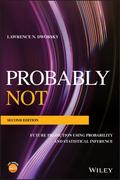John Wiley & Sons Probably NotA revised edition that explores random numbers, probability, and statistical inference at an introdu.. Product #: 978-1-119-51810-5 Regular price: \$85.62 \$85.62 Auf Lager

# Probably Not

## Future Prediction Using Probability and Statistical Inference2. Auflage September 2019
352 Seiten, Softcover
Wiley & Sons Ltd

ISBN: 978-1-119-51810-5
John Wiley & Sons

Jetzt kaufen

### Preis: 89,90 €

Preis inkl. MwSt, zzgl. Versand

A revised edition that explores random numbers, probability, and statistical inference at an introductory mathematical level

Written in an engaging and entertaining manner, the revised and updated second edition of Probably Not continues to offer an informative guide to probability and prediction. The expanded second edition contains problem and solution sets. In addition, the book's illustrative examples reveal how we are living in a statistical world, what we can expect, what we really know based upon the information at hand and explains when we only think we know something.

The author introduces the principles of probability and explains probability distribution functions. The book covers combined and conditional probabilities and contains a new section on Bayes Theorem and Bayesian Statistics, which features some simple examples including the Presecutor's Paradox, and Bayesian vs. Frequentist thinking about statistics. New to this edition is a chapter on Benford's Law that explores measuring the compliance and financial fraud detection using Benford's Law. This book:
* Contains relevant mathematics and examples that demonstrate how to use the concepts presented
* Features a new chapter on Benford's Law that explains why we find Benford's law upheld in so many, but not all, natural situations
* Presents updated Life insurance tables
* Contains updates on the Gantt Chart example that further develops the discussion of random events
* Offers a companion site featuring solutions to the problem sets within the book

Written for mathematics and statistics students and professionals, the updated edition of Probably Not: Future Prediction Using Probability and Statistical Inference, Second Edition combines the mathematics of probability with real-world examples.

LAWRENCE N. DWORSKY, PhD, is a retired Vice President of the Technical Staff and Director of Motorola's Components Research Laboratory in Schaumburg, Illinois, USA. He is the author of Introduction to Numerical Electrostatics Using MATLAB from Wiley.

Acknowledgments

Introduction

1 An Introduction to Probability

Predicting The Future

Rule Making

Random Events and Probability

The Lottery

Coin Flipping

The Coin Flip Strategy That Can't Lose

The Prize Behind The Door

The Checker Board

Problems

2 Probability Distribution Functions and Some Math Basics

The Probability Distribution Function

Averages and Weighted Averages

Expected Values

The Basic Coin Flip Game

PDF Symmetry

Standard Deviation

Cumulative Distribution Function

The Confidence Interval

Final Points

Rehash and Histograms

Problems

3 Building A Bell

Problems

4 Random Walks

The One-Dimensional Random Walk

Some Subsequent Calculations

Diffusion

Problems

5 Life Insurance

Introduction

Life Insurance

Insurance As Gambling

Life Tables

Birth Rates and Population Stability

Life Tables, Again

Social Security - Sooner Or Later?

Problems

6 The Binomial Theorem

Introduction

The Binomial Probability Formula

Permutations and Combinations

Large Number Approximations

The Poisson Distribution

Disease Clusters

Clusters

Problems

7 Pseudorandom Numbers and Monte -Carlo Simulations

Random Numbers and Simulations

Pseudo-Random Numbers

The Middle Square PRNG

The Linear Congruential PRNG

A Normal Distribution Generator

An Arbitrary Distribution Generator

Monte Carlo Simulations

A League of Our Own

Discussion

Notes

8 Some Gambling Games In Detail

The Basic Coin Flip Game

The "Ultimate Winning Strategy"

Parimutuel Betting

The Gantt Chart and A Hint of Another Approach

Problems

9 Scheduling and Waiting

Introduction

Scheduling Appointments In The Doctor's Office

Lunch with A Friend

Waiting for A Bus

Problems

10 Combined and Conditional Probabilities

Introduction

Functional Notation (Again)

Conditional Probability

Medical Test Results

The Shared Birthday Problem

Problems

11 Bayesian Statistics

Bayes Theorem

Multiple Possibilities

Will Monty Hall Ever Go Away?

Philosophy

The Prosecutor's Fallacy

Continuous Functions

Credible Intervals

Gantt Charts (Again)

Problems

12 Estimation Problems

The Number of Locomotives Problem

Number of Locomotives, Improved Estimate

Decision Making

The Light House Problem

The Likelihood Function

The Light House Problem II

Introduction

Another Parrondo Game

The Parrondo Ratchet

Problems

14 Benford's Law

Introduction

History

The 1/x Distribution

Goodness of Fit Measure

Smith's Analysis

Problems

15 Networks, Infectious Diseases and Chain Letters

Introduction

Degrees of Separation

Propagation Along The Networks

Some Other Networks

Neighborhood Chains

Chain Letters

16 Introduction To Frequentist Statistical Inference

Introduction

Sampling

Sample Distributions and Standard Deviations

Estimating Population Average From A Sample

The Student-T Distribution

Polling Statistics

Did A Sample Come From A Given Distribution?

A Little Reconciliation

Correlation and Causality

Correlation Coefficient

Regression Lines

Regression To The Mean

Problems

17 Statistical Mechanics and Thermodynamics

Introduction

Statistical Mechanics

(Concepts of) Thermodynamics

18 Chaos and Quanta

Introduction

Chaos

Probability In Quantum Mechanic

Appendix

Introduction

Continuous Distributions and Integrals

Exponential Functions

Index
LAWRENCE N. DWORSKY, PHD, is a retired Vice President of the Technical Staff and Director of Motorola's Components Research Laboratory in Schaumburg, Illinois, USA. He is the author of Introduction to Numerical Electrostatics Using MATLAB(r) from Wiley.

L. N. Dworsky, Motorola, Inc.